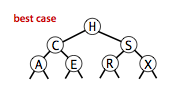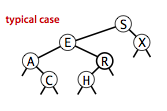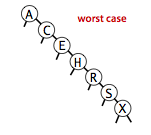# 二叉查找树

Wafer Li ... 2017-02-14 《Algorithm 第四版》笔记
• Algorithm
• 读书笔记

## # 1. 介绍

1. 每个节点都拥有一个 Comparable
2. 每个节点都大于它的左子节点，小于它的右子节点## # 2. 数据结构的实现

1. 左子结点的链接
2. 右子结点的链接
3. 以这个节点为根节点的树的节点总数
4. 节点的键和值
The Node of Binary Search Tree
Node leftChild Key key Value val int N Node rightChild

Code:

// The inner class of Node
private class Node {
private Key key;
private Value val;
private Node left, right;

// 以该节点为根的子树的节点个数，包括根节点
private int N;

public Node(Key key, Value val, int N) {
this.key = key;
this.val = val;
this.N = N;
}
}

1
2
3
4
5
6
7
8
9
10
11
12
13
14
15

## # 3. API 实现

1. get(), put()
2. min(), max(), floor(), ceiling()
3. delete(), deleteMin(), deleteMax()

### # 3.1 搜索

1. 如果根节点和所给的键值相等，那么命中
2. 如果所给的键值比根节点小，那么就在其左子树搜索
3. 如果所给的键值比根节点大，那么就在其右子树搜索
4. 假如最终找到了 null，那么说明所给键值不在符号表中，返回 null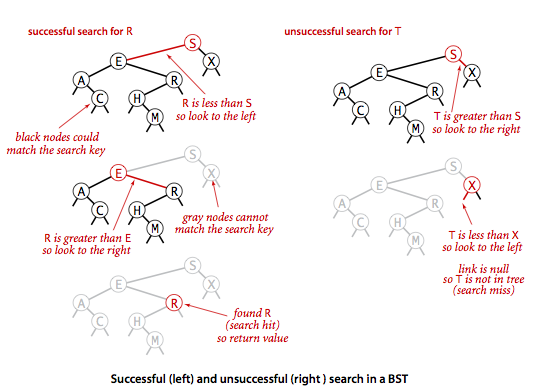Code:

public Value get(Key key) {
return get(root, key);
}

private Value get(Node x, Key key) {

if(x == null) {
return null;
}

int cmp = key.compareTo(x.key);

if(cmp < 0)
return get(x.left, key);
else if(cmp > 0)
return get(x.right, key);
else
return x.val;
}

1
2
3
4
5
6
7
8
9
10
11
12
13
14
15
16
17
18
19

### # 3.2 插入

1. 如果键值已经存在于符号表中，那么就更新它的值
2. 如果键值不在符号表中，那么就创建一个新的节点存储键值对

public void put(Key key, Value val) {
root = put(root, key, val);
}

private Node put(Node x, Key key, Value val) {

if (x == null)
return new Node(key, val, 1);

int cmp = key.compareTo(x.key);

if (cmp < 0)
x.left = put(x.left, key, val);
else if (cmp > 0)
x.right = put (x.right, key, val);
else
x.val = val;

// 更新节点个数
x.N = size(x.left) + size(x.right) + 1;
return x;

}

1
2
3
4
5
6
7
8
9
10
11
12
13
14
15
16
17
18
19
20
21
22
23

if (x == null) {
x = new Node(key, val);
}

1
2
3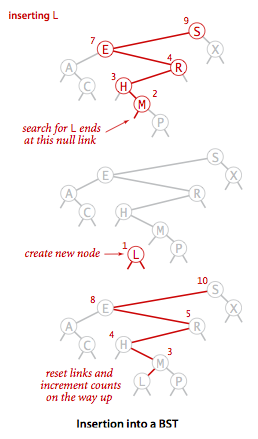### # 3.3 删除

#### # 3.3.1 删除 最小/最大 值

1. 一直深入左子树去查找，然后定位到最小的节点
2. 将它的右子树和它的父节点连接
3. 由于原有的连接最小节点的链接被其右子树占据，没有指向最小节点的引用，那么它就会被垃圾回收机制回收。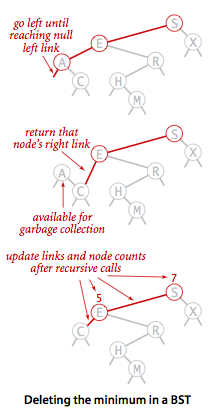public void deleteMin() {
root = deleteMin(root);
}

private Node deleteMin(Node x) {
if (x.left == null)
return x.right;

x.left = deleteMin(x.left);
x.N = size(x.left) + size(x.right) + 1;

return x;
}

1
2
3
4
5
6
7
8
9
10
11
12
13

put() 方法一样， delMin() 方法采用了递归的方法来深入树进行查找

#### # 3.3.2 通常节点的删除

1. x 保存一个副本 t

t 即所需要删除的元素

2. x 指向其后继 min(x.right)

后继即比 x 大的下一个节点，也就是其右子树中最小的节点 此时 x 已经指向后继。

3. x.right 指向 delMin(t.right)

这一步较为关键和难以理解。 delMin(t.right) 主要做了如下几件事：

1. 将后继从下层的链接释放出来

t 是原有 x 的副本，所以 delMin(t.right) 最后会查找到其后继，并释放出来。

2. 保持后继释放后的链接完整性。

delMin() 在查找到最小节点之后会返回右链接，由于调用递归性，右链接会被上层捕获，从而保持了链接性。 这里将后继释放出来后，会让其父节点来负责链接上其右子树。

3. 更新计数，并将传入的链接原样返回

这是最为重要的，首先将计数更新了，保证计数正确性。

其次，delMin(x) 在最后退出递归的时候会返回 x。 也就是 delMin(t.right) 的返回值是 t.right。 那么 x.right = delMin(t.right) 也就相当于 x.right = t.right

正好是使用了 x 来替代 t（注意此时 x 已经是后继了

4. x.left 指向 t.left

5. 更新计数器，递归方法返回传入值来保持链接完整性Code:

public void delete(Key key) {
root = delete(root,key);
}
private Node delete(Node x, Key key) {
// Notice that, we use recursive way
// to locate the Node which will be delete
// Also, we need to renew the counter

// Therefore, in this method, we need to
// retrun the link of the node itself, to
// miantain the connectivity

if (x == null) return null;
int cmp = key.compareTo(key);

// if not hit,
// deep into subtree and continue search
if (cmp < 0) x.left = delete (x.left, key);
else if (cmp > 0) x.right = delete(x.right, key);
else {  // hit
// The deletion of one or none child
if (x.right == null) return x.left;
if (x.left == null) return x.right;

// Two child
Node t = x;
x = min(t.right);
// refer to the special notice
x.right = deleteMin(t.right);
x.left = t.left;
}
// renew counter
x.N = size(x.left) + size(x.right) + 1;

return x;   // return itself to maintain the conectivity
}

1
2
3
4
5
6
7
8
9
10
11
12
13
14
15
16
17
18
19
20
21
22
23
24
25
26
27
28
29
30
31
32
33
34
35
36
37

### # 3.4 其他的有序方法

#### # 3.4.1 最小和最大

public Key min() {
return min(root).key;
}

private Node min(Node x) {
if (x.left == null) return x;
return min(x.left);
}

1
2
3
4
5
6
7
8

#### # 3.4.2 向下取整/向上取整

1. 如果所给的键值比根节点小，那么所需的节点就肯定在左子树中

2. 如果所给的键值比根节点大，那么所需的节点可能在右子树中

也就是说，如果我们在右子树中找不到所需节点，那么根节点就是所需节点

public Key floor(Key key) {
Node x = floor(root, key);
if (x == null) return null;
return x.key;
}

private Node floor(Node x, Key key) {
if (x == null) return null;
int cmp = key.compareTo(x.key);
if (cmp == 0) return x;
if (cmp < 0) return floor(x.left, key);
Node t = floor(x.right, key);
if (t != null) return t;
else return x;
}

1
2
3
4
5
6
7
8
9
10
11
12
13
14
15

#### # 3.4.3 选择和排序

select()rank() 是一对互逆方法，一个给排名，返回键值，另一个给键值，返回排名

1. 如果键值和根节点相等，那么根节点的左子树的总数就是所给节点的排名

2. 如果所给的键值比根节点小，那么就在左子树中寻找

3. 如果所给的键值比根节点大，那么在右子树中寻找，此时，所给节点的排名为：

$Rank = Size(left\ subtree) + Rank(right\ subtree) + 1$

左子树中的节点都比根节点小，既然所给节点都比根节点大，那么理应比左子树的节点都要大。所以排名还要加上左子树中的节点个数。其中的 $1$ 表示的是根节点本身。

public Key select(int k) {
return select(root, k).key;
}

private Node select(Nodex, int k) {
if (x == null) return null;
int t = size(x.left);
if (t >k) return select(x.left, k);
else if (t < k) return select(x.right,k - t - 1);
else return x;
}

public int rank(Key key) {
return rank(key, root);
}

private int rank(Key key, Node x) {
if(x == null) return  0;
int cmp = key.compareTo(x.key);
if (cmp < 0) return rank(key, x.left);
else if (cmp > 0) return 1 + size(x.left) + rank(key, x.right);
else return size(x.left);
}

1
2
3
4
5
6
7
8
9
10
11
12
13
14
15
16
17
18
19
20
21
22
23

#### # 3.4.4 范围

keys() 方法的主要算法思想就是要返回在一个特定范围内的所有键值

1. 如果根节点比范围小，那么找右子树

2. 如果根节点比范围大，那么找左子树

3. 如果根节点在范围内，将其入列，然后分别找左子树和右子树

这么做的目的是，如果一个节点在范围内，那么它的左子树和右子树也有可能在范围内

public Iterable<Key> keys() {
return keys(min(), max());
}

public Iterable <Key> keys(Key lo, Key hi) {
Queue<Key> queue = new Queue<Key>();
keys(root, queue, lo, hi);
return queue;
}

private void keys(Node x, Queue<Key> queue, Key lo, Key hi) {
if (x == null) return;
int comlo = lo.compareTo(x.key);
int cmphi = hi.compareTo(x.key);

// Notice that there is no *eles*,
// that's because we need to traversal the subtree
if (cmplo < 0) keys(x.left, queue, lo, hi);
if (cmplo <= 0 && cmphi >= 0) queue.enqueue(x.key);     // Within range
if (cmphi > 0) keys(x.right, queue, lo, hi);
}

1
2
3
4
5
6
7
8
9
10
11
12
13
14
15
16
17
18
19
20
21

## # 4. 性能

BST 的性能是依赖于输入模型的